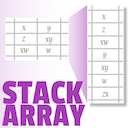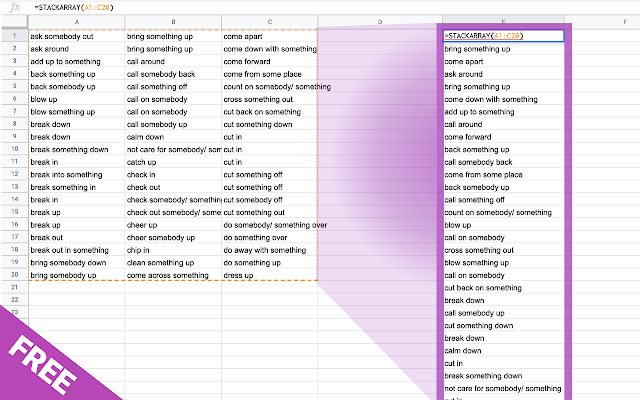Help & Support
Send FeedbackSTACKARRAY
Until now stacking many columns into a single one was a cumbersome process. Here is a formula seen in the web to stack columns with native Google Sheets function: =QUERY(ArrayFormula(SPLIT(TRANSPOSE(SPLIT(textjoin("-",TRUE,TRANSPOSE((A1:H1&","&A2:H5))),"-")),",")),"Select * where Col2<>''") Not very comprehensive, isn't it? Use =STACKARRAY(your_array) and stack your columns instantly wherever you need it in your spreadsheet. Without altering the initial array.
91 users
Works with
InstallOverview
```Until now combining many columns into a single one was a cumbersome process.
Here is a formula seen in the web to stack columns with native Google Sheets function:
=QUERY(ArrayFormula(SPLIT(TRANSPOSE(SPLIT(textjoin("-",TRUE,TRANSPOSE((A1:H1&","&A2:H5))),"-")),",")),"Select * where Col2<>''")

Not very comprehensive, isn't it?

Use =STACKARRAY(your_array) and stack your columns instantly wherever you need it in your spreadsheet. Without altering the initial array.

Use =STACKARRAY(your_array, TRUE) in order to remove all blank cells

To merge an array with only unique values, use =UNIQUE(STACKARRAY(your_array))
To sort the resulting column, use =SORT(STACKARRAY(your_array))```
Reviews